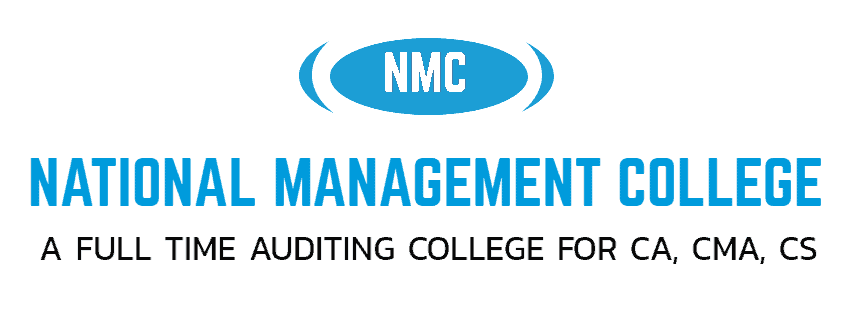# CA Foundation (Paper 3) Business Maths and Statistics 2

NATIONAL MANAGEMENT COLLEGE, THUDUPATHI.

PAPER – 3: BUSINESS MATHEMATICS, LOGICAL REASONING AND STATISTICS

Time Allowed : ½ hour                                               Maximum Marks: 25

1) Find the root of the equations. if  and

1. a) 2,1 b) -2,1 c)1,-2 d)1,2

2) The three roots of equation is.

(a) 1,-1,-9  (b) 1,-1,9

(c) 1,1,9  (d) -1,-1,-9

3) Find the value of K so that x =2 is a root of the equation 3x2 – 2kx + 5 = 0

(a) 17/4  (b) 4/17

(c) –17/4  (d) –4/17

4) The solutions of the set of inequations

a)( 24,0), ,(2,8),(0,12)      b)( 24,0), (2,8),(0,12) ,

1. c)(8, 4), (2, 8), (0, 12), (0, 24) c)(8,4), (0, 0) (0,6) (2,0)

5) Find the condition that one roots is double the other of ax2 +bx + c = 0

(a) 2b2 = 3ac (b) b2 = 3ac

(c) 2b2 = 9ac (d) 2b2 > 9ac

6) The solution set of the in equation x +2 > 0 and 2x – 6 > 0 is

1. a) b)                            c)                   d)

7) If the square of the number exceeds  twice of the number by 15 , then the number that satisfy the condition is

1. a) 5 b)3 c)-5 d) 15

8) The common region represented by the following in equalities

L1= X1 + X2    4;    L2 = 2X1 + X2   6

(a) OABC  (b) Outside of OAB

(c)  BCE  (d)  ABE

9) The highest degree of the linear equation is:

1. a) 0 b) 1 c) 2 d)4

10) The cost of two oranges and 3 apple is Rs.28  . If the cost of an apple is doubled then the cost of 3 oranges and 5 apples is Rs. 25  . The original cost of 7 oranges and 4 apple is ,

a)59 b)47 c)71 d) 63

11) On Solving the Inequalities 5x + y ≤ 100, x + y ≤ 60, x ≥ 0, y ≥ 0, we get the following situation:

(a) (0, 0), (20, 0), (10, 50) & (0, 60) (b) (0, 0), (60, 0), (10, 50) & (0, 60)

(c) (0, 0), (20, 0), (0, 100) & (10, 50)  (d) None of these

12) When two roots of quadratic equation are α,1/ α then what will be the quadratic equation:

(a) αx2 – (α2+1)x + α = 0 (b) αx2 – α2x+1 = 0

(c) αx2 – (α2+1)x + 1 = 0 (d) None of these

13) If 2x2 – (a + 6)2x + 12a – 0, then the roots are

(a) 6 and a (b) 4 and a2

(c) 3 and 2a  (d) 6 and 3a

14) Solving equation m + √��� = 6/25 the value of m works out to

(a) 1/25  (b) 2/25

(c) 3/25  (d) 1

15) Solve 4x+5 > 5x−13

1. a)x > -18 b)x > 5 or x < -13        c) x > -13 or x < 5     d) x > 18

16) Find the range of     if 1 < a < 3,0 < b < 2 and −2 < c < −1,

1. a) (-6,0) b)(0,6) c)( -3,0) d)(-6,-3)

17)  There are three cities: A,B and C. Three friends are discussing the population (in millions) of the three cities. One says A has 9 million people. The second says: B has as many people as A and C combined. The third says: The number of people in A added to half of the number of people in B is the number of people in C. What is the total number of people (in millions) in all three cities combined?

a)48 b)54 c)63 d)72

18)

.